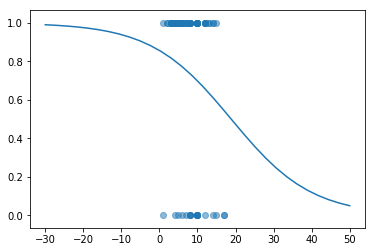## April 11: non-linear linear regression¶

[Prof. Mimno advising office hours 3-5 today!]

Previously we've discussed the intuition for linear models, how to fit models for one predictor, and algorithms for fitting models with multiple predictors. Today we'll discuss variations of linear models for data that doesn't fit a line.

1. Polynomial regession. Fit a curved output given only a single input. The trick: create multiple inputs that are functions of the output.

2. Logistic regession

Special Guest: Briana Vecchione on Datasheets for Datasets.

Of interest: Pandas data manipulation cheat sheet

The Stack Overflow developers survey

Open Data Mapathon for Disaster Relief & Food Security. Friday, April 12, 3 – 5pm, The Digital CoLab (Room 107) Olin Library. Pizza and drinks will be served.

In :
import pandas, numpy
from matplotlib import pyplot
import scipy.stats
from ipywidgets import interact, interactive, fixed, interact_manual
from sklearn.linear_model import LinearRegression, LogisticRegression


### Part 1: polynomial regression¶

We can expand our set of predictors by adding arbitrary funcitons of the inputs, and learning weights for those new predictors.

In :
curve_data = pandas.read_csv("curve.csv")


Can we fit curved data with a linear regression?

In :
pyplot.scatter(curve_data.x, curve_data.y)
pyplot.show()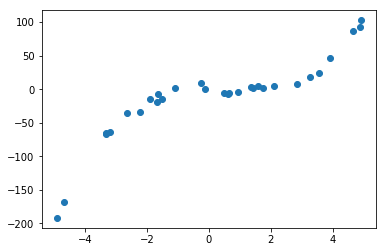In :
## Turn a single variable into three variables
def make_polynomial(x):
return pandas.DataFrame({"linear": x, "squared": x*x, "cubed": x*x*x})

In :
model = LinearRegression().fit(make_polynomial(curve_data.x), curve_data.y)
model.coef_

Out:
array([ 0.12850546, -1.90306821,  1.22714713])
In :
intervals = numpy.linspace(-5, 5, 30)
predictions = model.predict(make_polynomial(intervals))
pyplot.scatter(curve_data.x, curve_data.y)
pyplot.plot(intervals, predictions)
pyplot.show()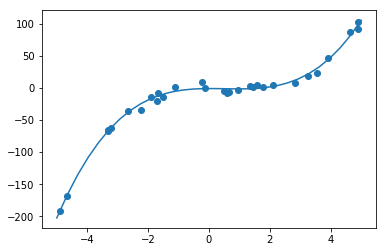In [ ]:
# Real data generating process:
#x = numpy.random.random(30) * 10 - 5
#y = 0.3 * x + -2 * x*x + 1.2 * x*x*x + numpy.random.normal(0, 5, 30)


### Part 2: Overfitting and polynomials¶

We have to keep track of the number of tunable parameters in our models. Could the model just be "memorizing" the input data?

In :
x = numpy.random.random(5)
y = numpy.random.random(5)

In :
polynomial_order = 4

high_order_poly = numpy.zeros((5,polynomial_order))
high_order_poly[:,0] = x
for i in range(1,polynomial_order):
high_order_poly[:,i] = x * high_order_poly[:,i-1]
print(high_order_poly)

model = LinearRegression().fit(high_order_poly, y)
print("squared residuals: {:.4f}".format(numpy.sum((y - model.predict(high_order_poly)) ** 2)))
print("total squared: {:.4f}".format(numpy.sum((y - y.mean()) ** 2)))

model.coef_

[[2.59758202e-01 6.74743235e-02 1.75270089e-02 4.55278433e-03]
[5.99952572e-01 3.59943088e-01 2.15948782e-01 1.29559027e-01]
[2.42521304e-02 5.88165830e-04 1.42642744e-05 3.45939044e-07]
[2.72796329e-01 7.44178369e-02 2.03009127e-02 5.53801444e-03]
[3.82831873e-01 1.46560243e-01 5.61079324e-02 2.14799048e-02]]
squared residuals: 0.0000
total squared: 0.4267

Out:
array([ -218.10373643,  1538.59820751, -3757.44384604,  2959.89715672])
In :
evenly_spaced = numpy.zeros((30, polynomial_order))
evenly_spaced[:,0] = numpy.linspace(0,1, 30)

for i in range(1,polynomial_order):
evenly_spaced[:,i] = evenly_spaced[:,0] * evenly_spaced[:,i-1]

prediction = model.predict(evenly_spaced)

In :
pyplot.scatter(x, y)
pyplot.plot(evenly_spaced[:,0], prediction)
pyplot.show()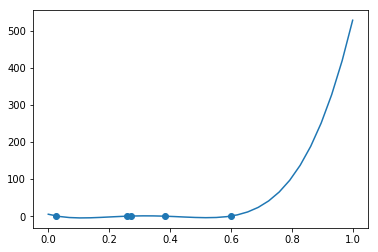### Part 3: Poisson regression¶

For linear regression, we want

$y = \beta_0 + \beta_1 x_1 + ... + \beta_K x_K$.

What if we want:

$y = e^{\beta_0 + \beta_1 x_1 + ... + \beta_K x_K} = e^{\beta_0} e^{\beta_1 x_1} ... e^{\beta_K x_K}$.

Alternatively,

$\log(y) = \beta_0 + \beta_1 x_1 + ... + \beta_K x_K$.

### Part 4: The logistic function¶

(Also called "sigmoid" and "softmax")

What is $e^x$ when $x$ is small, and big?

What is $\frac{e^x}{1+e^x}$?

In :
x_range = numpy.linspace(-8, 8, 30)
exp_x = numpy.exp(x_range)
pyplot.plot(x_range, exp_x / (1 + exp_x))
pyplot.show()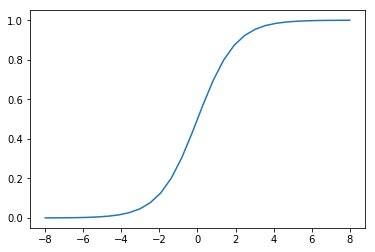What if we put a linear model inside a sigmoid?

$y = \frac{e^{b + mx}}{1 + e^{b + mx}}$

In :
def show_sigmoid(slope, intercept):
exp_x = numpy.exp(x_range * slope + intercept)
pyplot.plot(x_range, exp_x / (1 + exp_x))
pyplot.show()

interact(show_sigmoid, slope=(-2, 2, 0.1), intercept=(-5, 5, 0.1))

Out:
<function __main__.show_sigmoid>

### Part 5: Food preferences¶

In :
food = pandas.read_csv("food.csv")

Out:
Timestamp Nutella Age when you first tried Nutella Kale Age when you first tried Kale Tofu Age when you first tried Tofu Blue Cheese Age when you first tried Blue Cheese Cilantro Age when you first tried Cilantro
0 4/9/2019 11:37:52 4 8.0 3 19.0 3 6.0 4 16.0 NaN NaN
1 4/9/2019 11:38:22 4 6.0 4 10.0 2 12.0 1 10.0 NaN NaN
2 4/9/2019 11:38:42 4 12.0 4 9.0 1 18.0 3 12.0 4.0 10.0
3 4/9/2019 11:38:56 3 12.0 2 16.0 2 17.0 2 15.0 NaN NaN
4 4/9/2019 11:39:11 4 9.0 2 15.0 2 12.0 1 10.0 1.0 5.0
In :
food_frames = {}

for food_name in ["Nutella", "Kale", "Blue Cheese", "Cilantro"]:
age_var_name = "Age when you first tried {}".format(food_name)
frame = pandas.DataFrame({
"person": food.index,
"food": food_name,
"score": food[food_name],
"age": food[age_var_name]
})

frame = frame[ frame.age < 25 ]

food_frames[food_name] = frame.dropna()

In :
def logistic(x, model):
linear_output = x * model.coef_[0,0] + model.intercept_
exp_linear = numpy.exp(linear_output)
return exp_linear / (1 + exp_linear)

In :
frame = food_frames["Cilantro"]
pyplot.scatter(frame["age"], frame["score"], alpha=0.5)
pyplot.show()
frame["binary"] = frame.score.map(lambda x: 1.0 if x > 2 else 0.0)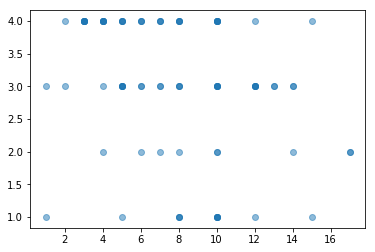In :
model = LogisticRegression().fit(frame[["age"]], frame["binary"])
print(model.coef_, model.intercept_)

[[-0.09480913]] [1.78835007]

In :
age_range = numpy.linspace(-30, 50, 30)
pyplot.scatter(frame["age"], frame["binary"], alpha=0.5)
pyplot.plot(age_range, logistic(age_range, model))
pyplot.show()